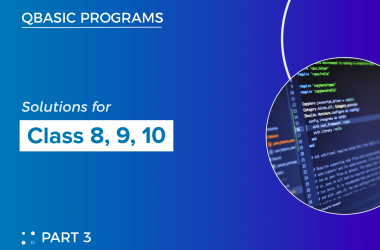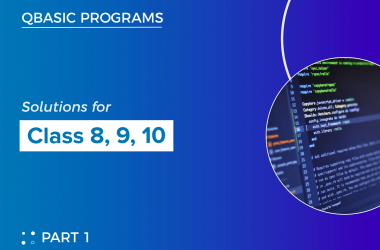# Functions in Qbasic:

A function is a built-in formula to accomplish a certain task such as mathematical, statistical, financial, logical/data calculations, etc. Functions in QBASIC are readymade programs that take some data, manipulate them and return the value, which may be a string or numeric type. Programming languages also have certain functions like spreadsheets or database software.

### TYPES OF FUNCTIONS: –

QBASIC supports two types of functions. They are: –

### A) USER-DEFINED FUNCTIONS: –

A function that is written and stored by the programmer to perform a specific task is known as User Defined Functions. When QBASIC does not provide a built-in function to solve any particular problem required by the programmer in that case only programmers define this type of function. FUNCTION……………END FUNCTION statement can be used to define the function. 

### B) BUILD-IN OR LIBRARY FUNCTIONS: –

The functions which are provided by the QBASIC system and also allow the programmer to use them according to the requirement are known as BUILT-IN or Library Functions. These functions are required to be called by the programmer to use them in a program. Some common Build-IN or Library Functions are LEN, LEFT\$, RIGHT\$, MID\$, UCASE\$, LCASE\$, CHR\$………etc.  Here are the types of library functions:

·   Mathematical Functions

The numerical data is processed using mathematical functions. The following are some of the mathematical functions used in QBASIC:

–        SQR Function

The SQR Function gives the result as the square root of the given number.

Syntax:SQR (number)

``````PRINT SQR (49)
``````

–        ABS Function

This function is used to return the absolute value of a number or we can convert negative numbers into positive.

syntax:ABS(number)

``````PRINT ABS (-5)
``````

–        MOD Operator

This operator gives the remainder that comes after dividing two given numbers. It is also considered an arithmetic operator.

Syntax: N1 MOD N2

``5 MOD 2``

–        COS, SIN, and TAN Function

These are trigonometric functions. They are used to find the cosine, sine, and tangent of the given angle.

Syntax: COS (angle), SIN (angle), TAN (angle)

–        INT Function

This Function is used to obtain the largest integer from the given number.

Syntax: INT (number)

``````Example:
CLS
Pi=22/7
Num = 5.9999999
PRINT PI
PRINT INT (pi)
END
``````

The CINT function gives results by rounding up the fractional portion. It supports only -32768 to +32767.

Syntax: CINT (number)

``````                  CLS
CINT (12.4278)
END
``````

·   String Function

The string functions are used to process string data. Here are some of the string functions used in QBASIC:

–        LEN Function

This function gives output as the length of a given string.

Syntax: LEN (string)

``````CLS
A\$ = “COMPUTER”
PRINT LEN (A\$)
END
``````

–        LEFT\$

This function retrieves the specified number of characters from the string’s left side.

Syntax: LEFT\$ (string, n)

``````              CLS
A\$ = “COMPUTER”
PRINT LEFT\$ (A\$, 5)
END
``````

–        RIGHT\$

This function retrieves the specified number of characters from the string’s right

Side.

Syntax: RIGHT\$ (string, n)

``````               CLS
A\$= “COMPUTER”
PRINT RIGHT\$ (A\$, 5)
END
``````

–        LCASE\$

This functions changes the capital letters of given function into small letters.

Syntax: LCASE\$ (string)

``````               CLS
A\$ = “COMPUTER”
PRINT LCASE\$ (A\$)
END
``````

–        UCASE\$

This function changes small letters of a given function into capital letters.

Syntax: UCASE\$

``````PRINT UCASE\$ (“computer”
``````

### A)Some more library functions along with their uses are listed below:

1). MID\$:

MID\$ function returns a specific number of characters from a string.

2). LEN:

LEN function returns the number of characters in a string or the number of bytes required by a variable.

3). LCASE\$:

LCASE\$ function converts all the uppercase characters in lowercase.

4). UCASE\$:

UCASE\$ function converts a string to uppercase.

5). ASC:

ASC function converts a character or a string variable to its corresponding ASC II code. Only the first character of a string is evaluated by the ASC function.

6). CHR\$:

CHR\$ function retrieves the single character represented by the ASC II number.

7). STR\$:

STR\$ function converts a string expression to its string representation.

8). VAL:

VAL function converts a string expression consisting of digits into a numeric value.

9). LTRIM\$:

LTRIM\$ function removes leading blanks from the left side of the spring expression.

10). RTRIM\$:

RTRI\$ function removes trailing blanks from the right side of the string expression.

11). STRING\$:

STRING\$ function is used with a PRINT statement to display a particular character a specific number of times.

12). INSTR:

INSTR function returns the position of the first occurrence of a string in another string.

13). DATE\$:

DATE\$ function returns the current system date of the computer and allows to set the current system date.

14). TIME\$:

TIME\$ function returns the current system time of the computer and allows to set the current time.

##### Previous Post## 1. MEASUREMENT OF SOUND PRESSURE LEVEL (SPL) IN DIFFERENT MICROENVIRONMENTAL SETTINGS

##### Related Posts## File Handling in Qbasic

In QBasic, you can use the OPEN and CLOSE statements to work with files. To open a file…## Qbasic programs solutions(part 3) for class 8, class 9 and class 10

Here, I have listed some of the qbasic programs. We also have mobile app where you can view…## Qbasic programs with solutions(part 1) for class 8, class 9 and class 10

Here, I have listed some of the qbasic programs. We also have mobile app where you can view…